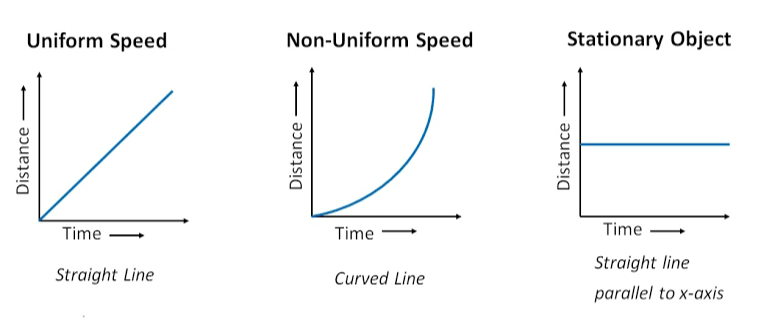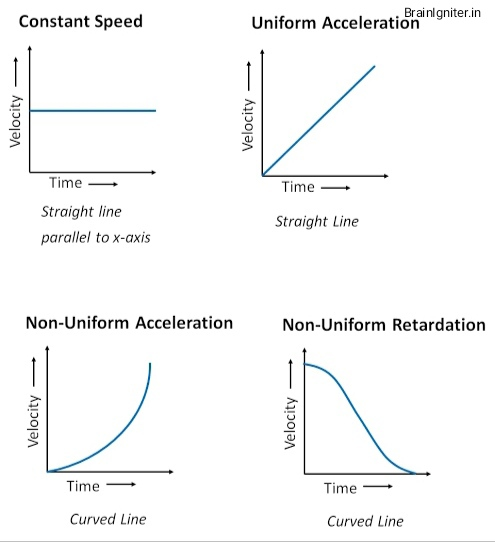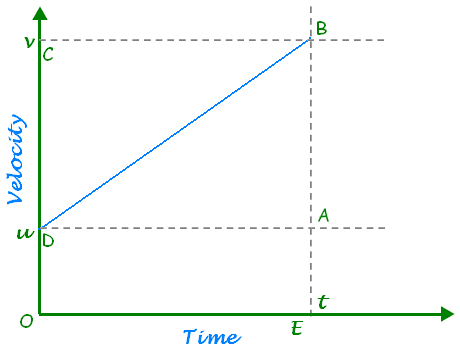# Class 9 Motion Notes

Motion Notes

## Motion:-

When a body changes its position with respect to time then the body is said to be in motion.

## Rest:-

A body is said to be in rest if it does not change its position with respect to time.

## Rectilinear Motion:-

Motion in a straight line is called rectilinear motion.

## Scalar Quantity:-

The physical quantity which depends only on magnitude is called scalar quantity. Ex:- distance, mass, speed etc.

## Vector Quantity:-

The physical quantity which depends on both magnitude and direction is called vector quantity. Ex:- displacement, velocity, weight, acceleration etc.

## Distance:-

The length of the actual path covered by an object is called distance.

• S.I. unit:- meter (m)
• It is a scalar quantity.

## Displacement:-

The shortest distance between initial and final point is called displacement.

• S.I. unit:- meter (m)
• It is a vector quantity.

## Uniform Motion:-

The motion of an object is said to be uniform if it covers equal distances in equal intervals of time.

## Non-uniform Motion:-

The motion of an object is said to be non-uniform if it does not cover equal distances in equal intervals of time.

## Speed:-

The distance covered by an object per unit time is called speed.

• Speed = Distance/Time
• S.I. unit:- meter/second
• Quantity:- Scalar
• Average speed = Total distance covered/Total time taken

## Velocity:-

The displacement of an object per unit time is called velocity.

• Velocity = Displacement/Time
• S.I. unit:- meter/second
• Quantity:- Vector
• Average velocity = (Initial velocity + Final velocity)/2 = (u + v)/2

## Acceleration(a):-

The change in velocity of an object per unit time is known as acceleration.

• a = (v – u)/t
• S.I. unit:- meter/second2 Or, m/s2
• Quantity:- Vector
• Odometer measures the distance covered by vehicles.
• Speedometer measures the speed of the vehicles.

## Distance – Time Graphs:-

.• The slope of distance – time graph gives the speed of the object.

## Velocity – Time Graphs:-• The area under the velocity-time graph gives the distance (magnitude of displacement).
• The slope of velocity – time graph gives the acceleration of the object.

## Equation For Velocity – Time Relation:-Consider an object moving with initial velocity ‘u’ and acceleration ‘a’. Let after time ‘t’, its final velocity is ‘v’.

From graph, OD = u, OC = v and OE = t

acceleration = Change in velocity/Time

or, a = DC/OE

or, a = (OC – OD)/OE

or, a =(v – u)/t

or, at = v – u

or, v = u + at

## Equation For Position – Time Relation:-Consider an object moving with initial velocity ‘u’ and acceleration ‘a’. Let after time ‘t’, its final velocity is ‘v’.

From graph, OD = u, OC = BE = v and OE = t

Distance traveled = area of trapezium ODBE

or, S = 1/2(sum of parallel sides) x distance between them

or, S = 1/2(OD + BE) x OE

or, S = 1/2(u + v) x t

or, S = 1/2(u + u + at) x t [since, v = u + at]

or, S = 1/2(2u + at) x t

or, S = ut + (1/2)at2

## Equation For Position – Velocity Relation:-Consider an object moving with initial velocity ‘u’ and acceleration ‘a’. Let after time ‘t’, its final velocity is ‘v’.

From graph, OD = u, OC = BE = v and OE = t

Distance traveled = area of trapezium ODBE

or, S = 1/2(sum of parallel sides) x distance between them

or, S = 1/2(OD + BE) x OE

or, S = 1/2(u + v) x t

or, S = 1/2(u + v)(v – u)/a [since, v = u + at So, t = (v – u)/a]

or, S = (v2 – u2)/2a

or, 2aS = v2 – u2

## Uniform circular motion:-

When a body moves in a circular path with uniform speed, its motion is called uniform circular motion.

Note:- Circular motion is always an accelerated motion because the object changes its direction at every point of its motion.